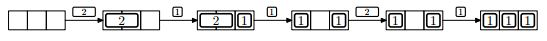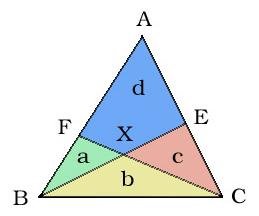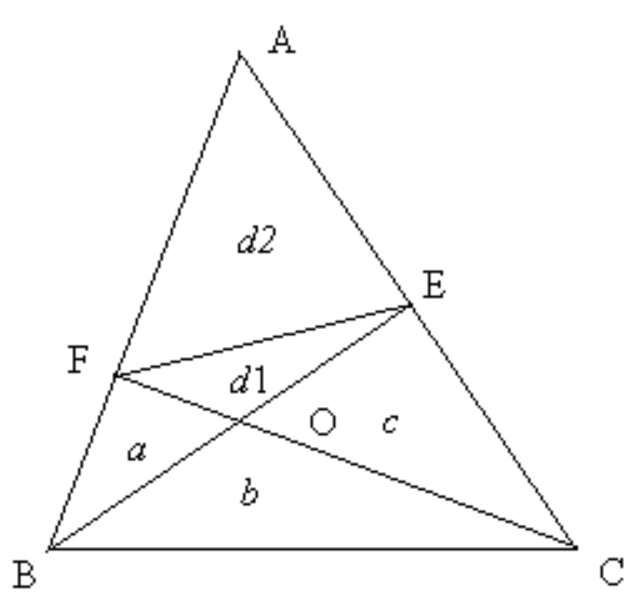e-olymp 8678. Birches

The State National Park $Q$ recently acquired a beautiful birch avenue consisting of $n$ trees. Each tree has a height of $H_i$.

International Classification of national parks is a list of the most beautiful nature reserves in the world. Used to rank parks such a thing as $distinctiveness$ which is understood as the number of pairs ($i$, $j$), for which the observed ratio of $H_i$ $mod$ $H_j$ = $k$, where $k$ is a special number, which is selected by the Expert Council of the international organization of national parks.

What $distinctiveness$ has national park state $Q$?

Input

The first line has two positive integers $n$ and $k$ $\left(1\leqslant n\leqslant 10^5, 0\leqslant k\leqslant 10^6\right)$ — the number of trees in the national park and a special number of the advisory council, respectively.

The second line has $n$ numbers $H_i$ $\left(1\leqslant H_i\leqslant 10^6\right)$ — the height of the trees in the park.

Output

In the single line print $Q$ national park $distinctiveness$.

Tests

 № Input Output 1 5 1 1 2 3 4 5 8 2 6 2 2 6 7 8 10 14 8 3 15 6 1 4 5 6 9 13 15 16 19 20 2124 27 45 49 14 4 7 3 1 5 7 8 9 23 46 2 5 10 15 23 26 67 79 82 110 118 200 450 900 2

Solution

To solve this problem, we will count the number of identical elements, while entering the array. Next, if $i$ and $j$ were met more than $0$ times and $i$ is not equal $j$, we add the counter x + = cnt [j] * cnt [i], and if $i$ = $j$ — x + = cnt [i] * (cnt [i] - 1).

Задача

Маленький Вася играет в новую компьютерную игру — пошаговую стратегию «Энергетический магнат».

Правила игры достаточно просты:

• Доска содержит $n$ слотов, расположенных в линию.
• Имеется набор электростанций, каждая из которых занимает один или два слота подряд, и производит одну единицу энергии.
• Каждый ход игры позволяет построить одну новую электростанцию, ее можно расположить на доске в любом месте по Вашему усмотрению. Если для новой электростанции нет места, Вы можете удалить некоторые старые электростанции.
• После выполнения каждого хода компьютер вычисляет количество энергии, производимой расположенными на доске электростанциями, и добавляет его к общему количеству очков в игре.Пример №1.

Вася уже знает все типы электростанций, которые он сможет построить на каждом шаге игры. Он хочет знать, какое максимальное количество очков он сможет получить в игре. Можете ли Вы ему помочь?

Входные данные

Первая строка содержит число $n \left(1\leqslant n\leqslant 1000 \right)$ — количество слотов на доске. Вторая строка содержит строку $s$. $i$-ый символ строки равен $1$, если Вы можете построить однослотовую электростанцию в $i$-ом раунде, и символ $2$, если Вы можете построить двуслотовую электростанцию в $i$-ом раунде. Количество раундов в игре не превосходит $100000$.

Выходные данные

Вывести одно число — наибольшее количество очков, которое можно достичь.

Тесты

 № Вхлодные данные Выходные данные 1 3 21121 10 2 2 12 2 3 2 211 4

Решение задачи

Будем увеличивать num на 1, если встречается двуслотовая электростанция. Если нет свободных слотов $temp — c\lt0$ и $num\geqslant1$, то вместо двуслотовой ставим станцию, которая в этом ходе должна быть $temp += (2 — c)$ и вычитаем одно очко. В итоге смотрим, если двуслотовых электростанций нет $num\gt 1$, то считаем однослотовые: $n — temp$, а если они есть, то: $n — temp — num$.

Ссылки

• Условие задачи на e-olymp
• Код программы ideone

ЗадачаКороль страны Геометрии в заботах. У него есть три сына, которые постоянно ссорятся. Король применял разные методы примерения, но все напрасно. И это его очень беспокоило.

«А что если разделить королевство?» подумал король. Он пригласил советников и описал свой план. Король открыл карту.

Королевство имеет форму треугольника с вершинами $A, B, C$. Король провел линию от $B$ к $E$ ($E$ — произвольная точка на отрезке $AC$) и линию от $C$ к $F$($F$ — произвольная точка на отрезке $AB$). Пересечение $BE$ и $CF$ обозначено через $X$.

Теперь образовалось четыре части — $a$ (треугольник $BFX$), $b$ (треугольник $BCX$), $c$ (треугольник $CEX$) и $d$ (четырехугольник $AEXF$). Король решил отдать области$a$, $b$, $c$ трем сыновьям. А область $d$ станет новым королевством.

Вы — главный советник. Король сообщает Вам значения $a, b, c$. Вам необходимо найти значение $d$. Если его найти невозможно, то сообщить об этом.

Входные данные

Состоит из не более чем $1000$ тестов. Каждый тест содержит три неотрицательных действительных числа $a$, $b$, $c$ (разделенных пробелом). Входные данные заканчиваются тестом у которого $a = -1$ и он не обрабатывается.

Выходные данные

Для каждого теста вывести его номер, начиная с $1$. В следующей строке вывести $d$ (величина области королевства после раздела) округленное до $4$ десятичных знаков или ‘Poor King!’ (без кавычек) если значение $d$ определить невозможно. Формат выходных данных показан в примере.

Тесты

 № Входные данные Выходные данные 1 1 2 1 Set 1: 2.0000 2 2 4 2 Set 2: 4.0000 3 1 3 3 Set 3: 5.0000 4 -1 0 0

Решение задачиДля решения задачи соединим точки  $F$ и $E$ линией. Получили два треугольника: $d1$ и $d2$. Искомую площадь $d$ будем искать как сумму площадей $d1$ и $d2$. Треугольники $BFO$ и $EFO$ имеют общее основание $FO$. Следовательно их площади $d1$ и $a$ относятся как высоты, опущенные из вершин $E$ и $B$ на прямую $FO$. Аналогично треугольники $BCO$ и $ECO$ имеют общее основание $OF$. Значит их площади $c$ и $b$ относятся как высоты, опущенные из вершин $E$ и $B$ на прямую $CO$. То есть $\frac{d_1}{a}=\frac{c}{b}$. Отсюда $d_1=\frac{ac}{b}$. Теперь рассмотрим треугольники $CAF$ и $CBF$ с основаниями $AF$ и $BF$. Они имеют одинаковую высоту, опущенную из вершины $С$ на прямую $AB$. Следовательно площади этих треугольников относятся как длины сторон $AF$ и $BF$. Аналогично треугольники $EAF$ и $EBF$ имеют основания $AF$ и $BF$. Они имеют одинаковую высоту, опущенную из вершины $E$ на прямую $AB$. Площади этих треугольников относятся как длины сторон $AF$ и $BF$. Тогда $$\frac{AF}{BF}=\frac{S_{\blacktriangle} CAF}{S\blacktriangle CBF}=\frac{c+d_1+d_2}{a+b}$$. $$\frac{AF}{BF}=\frac{S\blacktriangle EAF}{S\blacktriangle EBF}=\frac{d_2}{a+d_1}$$. Следовательно $\frac{c+d_1+d_2}{a+b}=\frac{d_2}{a+d_1}$. Поскольку $d1$ уже найдено, то имеем равенство с одним неизвестным $d2$ : $$d_2=\frac{(c+d_1)(a+d_1)}{b-d_1}$$. Если $b \leqslant d1$, то решения не существует.

Ссылки

• Условие задачи на e-olymp
• Код программы на ideone

ЗадачаПосле окончания второго тура олимпиады по программированию участники олимпиады решили отметить это событие. Для этой цели был заказан один большой торт прямоугольной формы. При этом стол, вокруг которого собрались участники был круглым. Естественно, у них возник вопрос, поместиться ли прямоугольный торт на круглом столе так, чтобы ни одна часть торта не выходила за пределы стола. Вам необходимо дать ответ на этот вопрос, зная размеры торта и радиус стола.

Входные данные

Содержит три натуральных числа: радиус стола $r \left(1\leqslant r\leqslant 1000 \right)$, ширину $w$ и длину $l$ торта $\left(1\leqslant w \leqslant l \leqslant 1000\right)$.

Выходные данные

Вывести слово $YES$, если торт помещается на стол, и слово $NO$ в противном случае.

Тесты

 № Входные данные Выходные данные 1 38 40 60 YES 2 35 20 70 NO 3 50 60 80 YES 4 30 60 90 NO

с ветвлением:

без ветвления:

Решение задачиВписанный в окружность прямоугольник

Для того, чтобы узнать, помещается торт на столе или нет, необходимо найти диагональ прямоугольного торта. Зная длину и ширину прямоугольника, находим диагональ по теореме Пифагора. Если она равна или меньше диаметра стола $AB^2$ + $AD^2$ <= 4$OD^2$, значит торт помещается, и пишем  "YES". Если диагональ больше диаметра стола, пишем  "NO".

Ссылки

• Условие задачи на e-olymp
• Код программы с ветвлением на ideone
• Код программы без ветвления на ideone